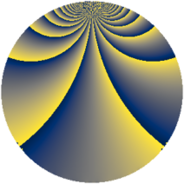Properties

 Label 784.2.bbLevel $784$ Weight $2$ Character orbit 784.bb Rep. character $\chi_{784}(111,\cdot)$ Character field $\Q(\zeta_{14})$ Dimension $168$ Newform subspaces $2$ Sturm bound $224$ Trace bound $1$

Related objects

Defining parameters

 Level: $$N$$ $$=$$ $$784 = 2^{4} \cdot 7^{2}$$ Weight: $$k$$ $$=$$ $$2$$ Character orbit: $$[\chi]$$ $$=$$ 784.bb (of order $$14$$ and degree $$6$$) Character conductor: $$\operatorname{cond}(\chi)$$ $$=$$ $$196$$ Character field: $$\Q(\zeta_{14})$$ Newform subspaces: $$2$$ Sturm bound: $$224$$ Trace bound: $$1$$ Distinguishing $$T_p$$: $$3$$

Dimensions

The following table gives the dimensions of various subspaces of $$M_{2}(784, [\chi])$$.

Total New Old
Modular forms 708 168 540
Cusp forms 636 168 468
Eisenstein series 72 0 72

Trace form

 $$168 q - 28 q^{9} + O(q^{10})$$ $$168 q - 28 q^{9} + 16 q^{21} + 52 q^{25} + 60 q^{29} - 20 q^{37} - 4 q^{49} - 24 q^{53} + 8 q^{57} - 28 q^{61} - 24 q^{65} + 168 q^{69} + 24 q^{77} - 88 q^{81} - 24 q^{85} + 40 q^{93} + O(q^{100})$$

Decomposition of $$S_{2}^{\mathrm{new}}(784, [\chi])$$ into newform subspaces

Label Dim. $$A$$ Field CM Traces $q$-expansion
$a_{2}$ $a_{3}$ $a_{5}$ $a_{7}$
784.2.bb.a $48$ $6.260$ None $$0$$ $$0$$ $$0$$ $$0$$
784.2.bb.b $120$ $6.260$ None $$0$$ $$0$$ $$0$$ $$0$$

Decomposition of $$S_{2}^{\mathrm{old}}(784, [\chi])$$ into lower level spaces

$$S_{2}^{\mathrm{old}}(784, [\chi]) \cong$$ $$S_{2}^{\mathrm{new}}(196, [\chi])$$$$^{\oplus 3}$$